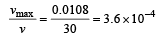Courses

# 28 Year NEET Questions: Waves- 3

## 30 Questions MCQ Test Physics 31 Years NEET Chapterwise Solved Papers | 28 Year NEET Questions: Waves- 3

Description
This mock test of 28 Year NEET Questions: Waves- 3 for NEET helps you for every NEET entrance exam. This contains 30 Multiple Choice Questions for NEET 28 Year NEET Questions: Waves- 3 (mcq) to study with solutions a complete question bank. The solved questions answers in this 28 Year NEET Questions: Waves- 3 quiz give you a good mix of easy questions and tough questions. NEET students definitely take this 28 Year NEET Questions: Waves- 3 exercise for a better result in the exam. You can find other 28 Year NEET Questions: Waves- 3 extra questions, long questions & short questions for NEET on EduRev as well by searching above.
QUESTION: 1

### Equation of a progressive wave is given by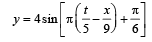Then which of the following is correct? 

Solution:

The standard equation of a progressive wave is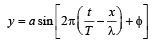The given equation can be written as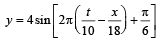∴ a = 4 cm, T = 10 s, λ = 18 cm and φ = π/6

Hence, (b) is correct.

QUESTION: 2

### The velocity of sound in any gas depends upon

Solution:

Velocity of sound in any gas depends upon density and elasticity of gas.

QUESTION: 3

### If the amplitude of sound is doubled and the frequency is reduced to one fourth, the intensity of sound at the same point will be 

Solution:

Intensity is proportional to (amplitude)2 and also intensity α (frequency)2. Therefore, intensity becomes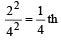QUESTION: 4

A 5.5 metre long  string has a mass of 0.035 kg. If the tension in the string is 77N, the speed of a wave on the string is 

Solution: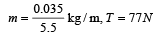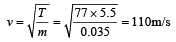QUESTION: 5

Velocity of sound waves in air is 330 m/s. For a particular sound wave in air, a path difference of 40 cm is equivalent to phase difference of 1.6π.The frequency of this wave is 

Solution:

From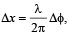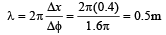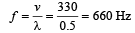QUESTION: 6

The transverse wave represented by the equation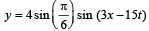has

Solution:

Compare the given equation with standard form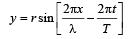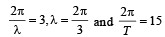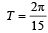Speed of propagation,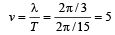QUESTION: 7

A closed organ pipe (closed at one end) is excited to support the third overtone. It is found that air in the pipe has 

Solution:

Third overtone has a frequency 7 n, which
means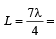three full loops + one half loop, which would make four nodes and four antinodes.

QUESTION: 8

Two trains move towards each other with the same speed. The speed of sound is 340 m/s. If the height of the tone of the whistle of one of them heard on the other changes 9/8 times, then the speed of each train should be 

Solution:

Here,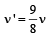Source and observer are moving in opposite direction, therefore, apparent frequency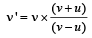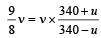⇒ 9 x 340 - 9u = 8 x 340 + 8u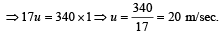QUESTION: 9

With the propagation of a longitudinal wave through a material medium, the quantities transmitted in the propagation direction are

Solution:

With the propagation of a longitudinal wave, energy alone is propagated.

QUESTION: 10

The frequency of sinusoidal wave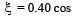[2000 t + 0.80] would be

Solution:

Comparing with the equation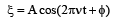We get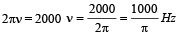QUESTION: 11

For production of beats the two sources must have

Solution:

For production of beats different frequencies are essential. The different amplitudes affect the minimum and maximum amplitude of the beats and different phases affect the time of occurrence of minimum and maximum.

QUESTION: 12

The temperature at which the speed of sound becomes double as was at 27°C is 

Solution:

We have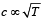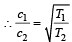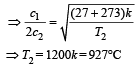QUESTION: 13

A stretched string resonates with tuning fork frequency 512 Hz when length of the string is 0.5 m. The length of the string required to vibrate resonantly with a tuning fork of frequency 256 Hz would be 

Solution: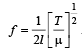When f is halved, the length is doubled.

QUESTION: 14

A standing wave is represented by y = Asin (100t) cos (0.01x), where y and A are in millimetre, t in seconds and x is in metre. Velocity of wave is 

Solution:

The wave equation is y = Asin (ωt)cos (kx); c = ω/k = 100/0.01 = 104 m/s.

QUESTION: 15

Which of the following equations represent a wave?

Solution:

y = A sin (at – bx + c) represents a wave, where a may correspond to ω and b may correspond to K.

QUESTION: 16

A wave of frequency 100 Hz is sent along a string towards a fixed end. When this wave travels back after reflection, a node is formed at a distance of 10 cm from the fixed end of the string. The speeds of incident (and reflected) waves are 

Solution:

As fixed end is a node, therefore, distance between two consecutive nodes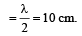λ = 20 cm = 0.2 m

As v = nλ    ∴ v = 100 x 0.2 = 20m/s

QUESTION: 17

From a wave equation: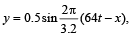the frequency of the wave is 

Solution: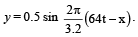Standard equation of the wave is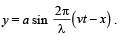Comparing the given equation with the standard equation, we get v = 64 and λ = 3.2.
Therefore, frequency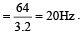QUESTION: 18

Two waves are approaching each other with a velocity of 20 m/s and frequency n. The distance between two consecutive nodes is 

Solution:

Distance between two successive nodes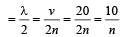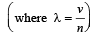QUESTION: 19

Two waves are said to be coherent, if they have 

Solution:

The waves, whose frequencies, phases and amplitudes are same at a given time or at a given place in space are known as coherent waves.

QUESTION: 20

The speed of a wave in a medium is 760 m/s. If 3600 waves are passing through a point in the medium in 2 min, then their wavelength is 

Solution:

Speed of the wave (v) = 760 m/s; Number of waves = 3600 and time taken (t) = 2 min = 120 sec. Frequecny of waves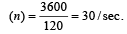.

∴ wavelength of waves  (λ)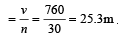[Alt :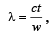where c = velocity of wave t = time in second w = no. of waves]

QUESTION: 21

A hospital uses an ultrasonic scanner to locate tumours in a tissue. The operating frequency of the scanner is 4.2 MHz. The speed of sound in a tissue is 1.7 km/s. The wavelength of sound in tissue is close to 

Solution:

Frequency (n) = 4.2 MHz = 4.2 × 106 Hz and speed of sound (v) = 1.7 km/s = 1.7 × 103 m/ s. Wave length of sound in tissue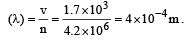QUESTION: 22

A source of sound gives 5 beats per second, when sounded with another source of frequency 100/sec. The second harmonic of the source, together with a source of frequency 205/sec gives 5 beats per second. What is the frequency of the source? 

Solution:

Frequency of first source with 5 beats/ sec = 100 Hz and frequency of second source with 5 beats/sec = 205 Hz. The frequency of the first source = 100 ± 5 = 105 or 95 Hz. Therefore, frequency of second harmonic of source = 210 Hz or 190 Hz. As the second harmonic gives 5 beats/ second with the sound of frequency 205 Hz, therefore, frequency of second harmonic source should be 210 Hz or frequency of source = 105 Hz.

QUESTION: 23

Two sound waves having a phase difference of 60º have path difference of 

Solution:

Phase difference =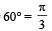Path difference =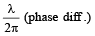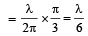QUESTION: 24

A star, which is emitting radiation at a wavelength of 5000 Å, is approaching the earth with a velocity of 1.50 × 106 m/s. The change in wavelength of the radiation as received on the earth is 

Solution:

Given : Wavelength (λ) = 5000 Å velocity of star (v) = 1.5 × 106 m/s.
We know that wavelength of the approaching star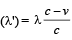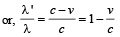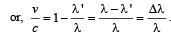Therefore,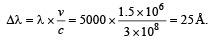[where Δλ = Change in the wavelength]

QUESTION: 25

What is the effect of humidity on sound waves when humidity increases? 

Solution:

Velocity of sound =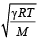When water vapour are present in air, average molecular weight of air decreases and hence velocity increases.

QUESTION: 26

The equation of a sound wave is given as: y = 0.0015 sin (62.4 x + 316 t). The wavelength of this wave is 

Solution:

y = 0.0015 sin (62.4x + 316t)

On comparing with y = A sin (ωt + kx)

ω = 316, k = 62.4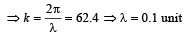QUESTION: 27

Two waves of the same frequency and intensity superimpose each other in opposite phases.After the superposition, the intensity and frequency of waves will 

Solution:

We know that interference is said to be constructive at point where resultant intensity is maximum (are in phase) and destructive at points where resultant intensity is minimum or 0 (are in opposite phase). Therefore, after the superposition, frequency and intensity of waves will remain constant.

QUESTION: 28

An organ pipe P1 closed at one end vibrating in its first overtone and another pipe P2, open at both ends vibrating in its third overtone are in resonance with a given tuning fork. The ratio of lengths of P1 and P2 respectively are given by

Solution:

We know that the length of pipe closed at one end for first overtone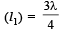and length of the open pipe for third overtone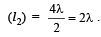Therefore, the ratio of
lengths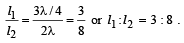QUESTION: 29

The speed of a wave in a medium is 960 m/s. If 3600 waves are passing through a point in the medium in 1 min., then the wavelength of the wave is 

Solution:

Given speed of wave (v) = 960m/s

Frequency of wave (f) = 3600/ min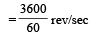= 60 rev per sec.

Wavelength of waves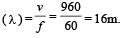[Alt :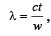where c = velocity of wave  t = time in second w = no. of waves]

QUESTION: 30

The equation of a travelling wave is y = 60 cos (180 t – 6x) where y is in microns, t in second and x in metres.The ratio of maximum particle velocity to velocity of wave propagation is 

Solution:

y = 60 cos (180t – 6x)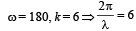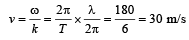Differentiating (1) w.r.t. t,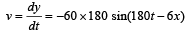vmax = 60 x 180μm/s

= 10800 μm/s = 0.0108 m/s